# Equivalence with Fractions

Before these lessons on equivalence with fractions the child needs to have had lots of time making fractions.

Have the child take a whole and find other fractions that equal the whole. If you have the fraction frames they can do this by putting the number inside the frames. If you have a box of loose fractions you could have the child experiment by placing the fraction pieces on top of a whole or by drawing around a whole on paper and then inserting various fractions into the whole.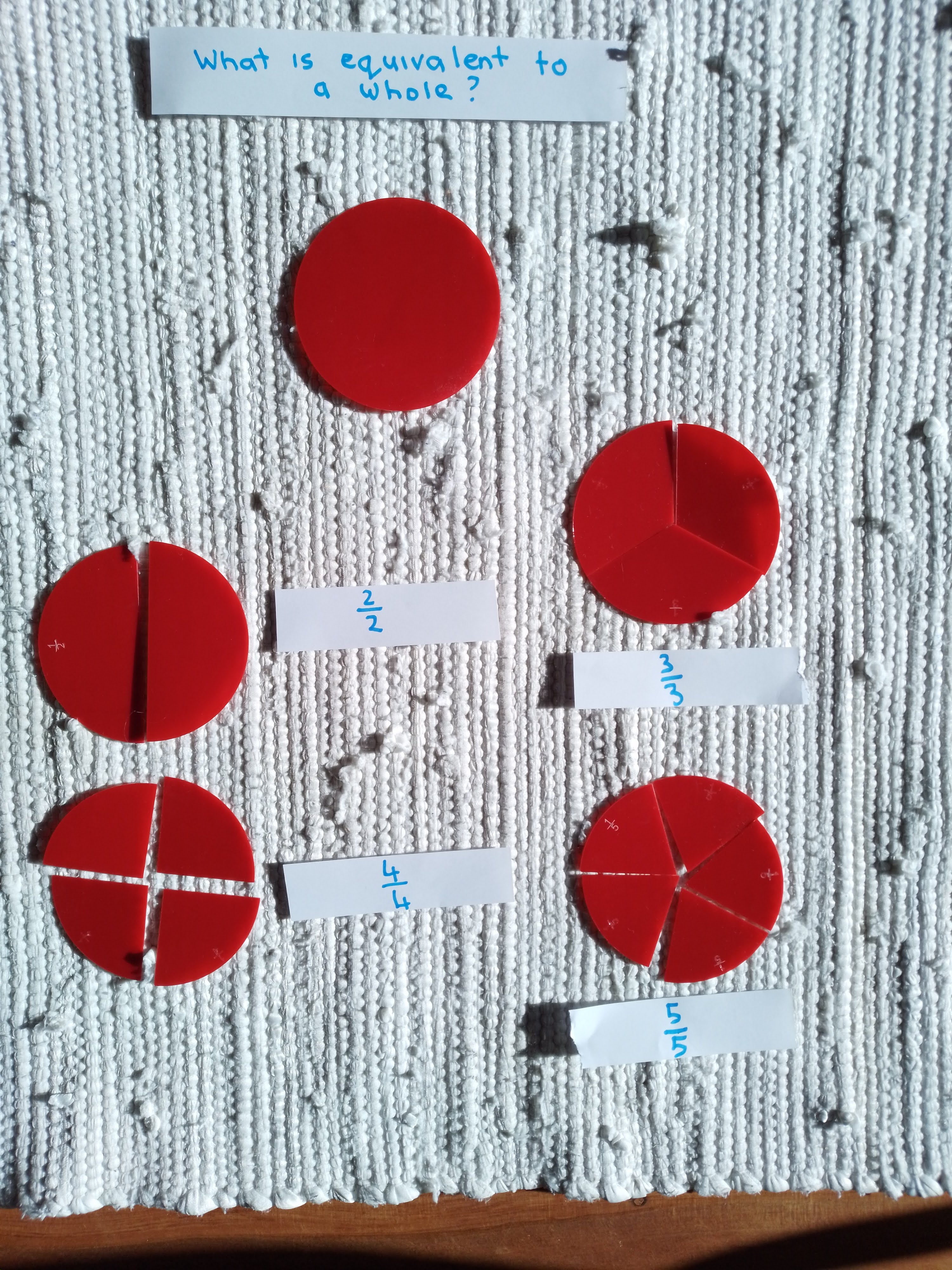Here the fractions are labelled to show the equivalence.

We drew around the fractions and coloured them using the bead bar colouring.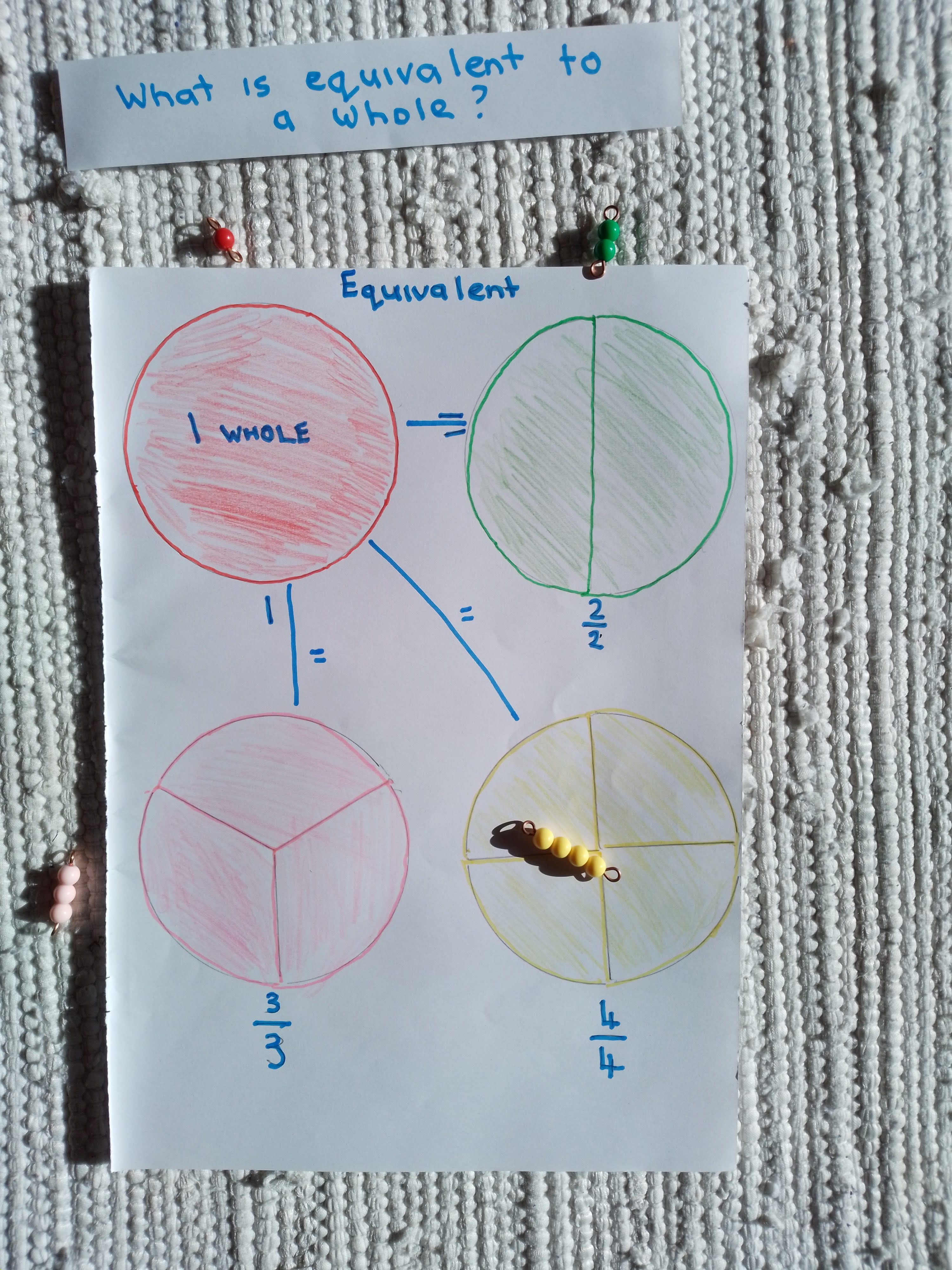The child could then spend time working with equivalences. The first set of cards in the Equivalent Fraction Task Cards provides opportunity for the child to explore with cards that have answers on the back.Presentation 2

Once the child has had a lot of time working with equivalences with a whole. Have a student take a half and find fractions that equal a half.

This could be done by drawing a half on paper with the fraction circle and then finding through trial and error fraction pieces that equal a half.

Students could write equations to show the equivalences and if appropriate write these into a book.  You'll see in this example I've changed the traditional order of the equation. You can read more about why here.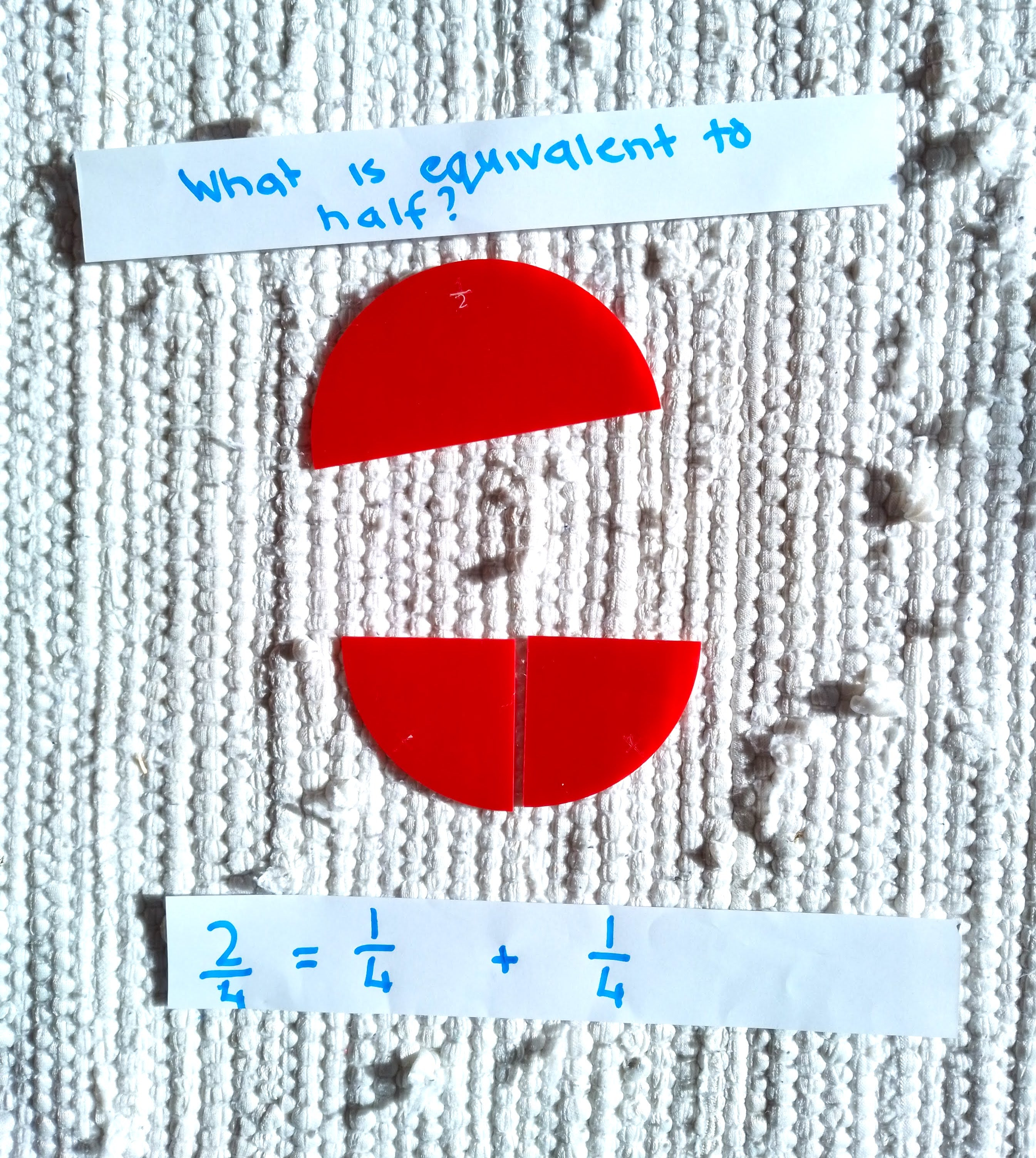The second two set of cards in the Equivalent Fraction Task Cards provide opportunity for the child to explore with 40 task cards that have answers on the back.Your child might also like this equivalent fraction booklet which is free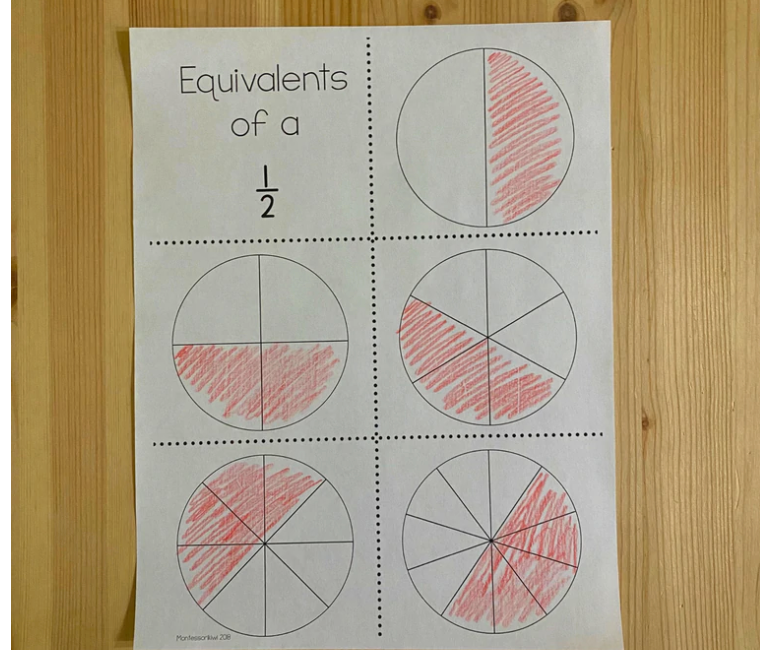PIN IT FOR LATER: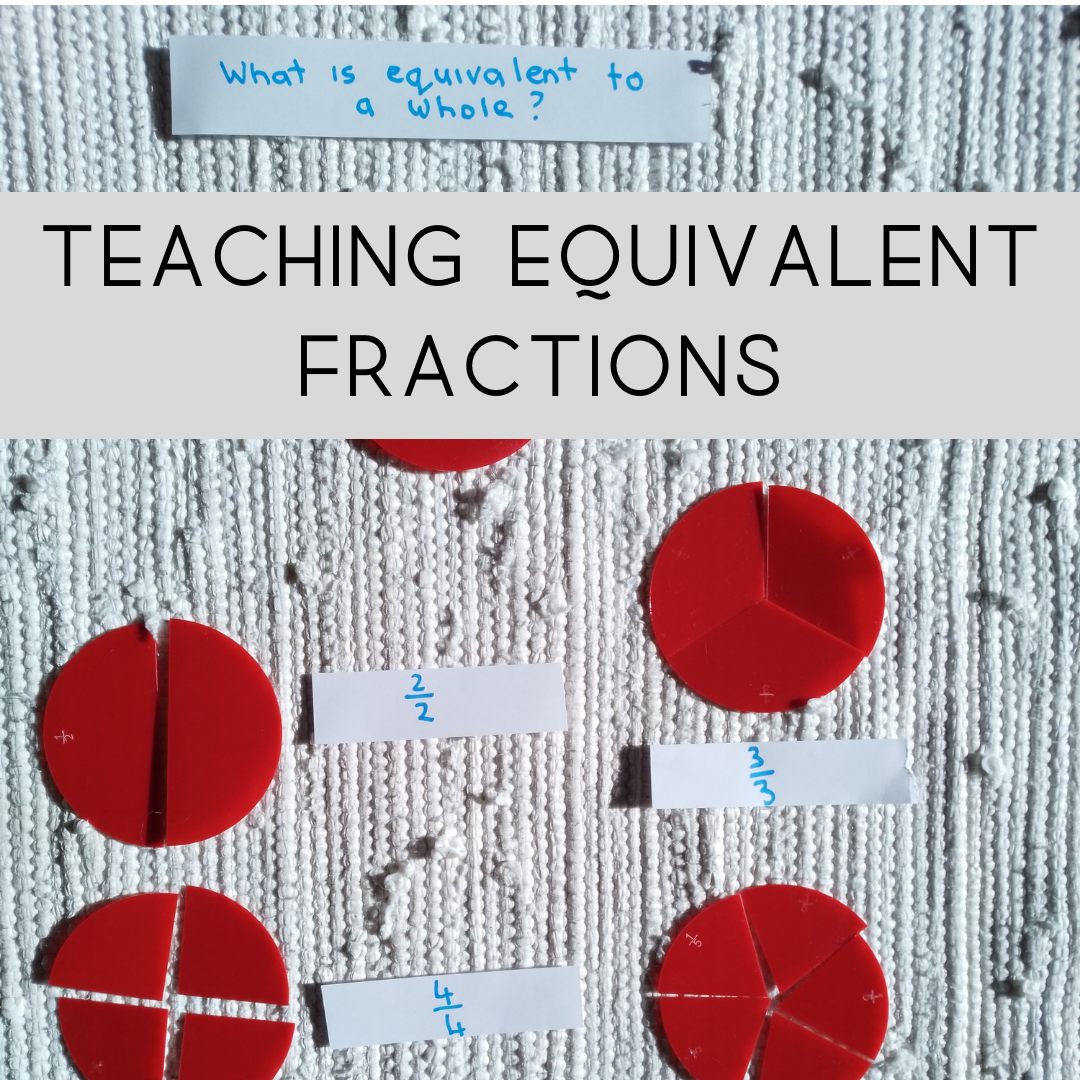Interested in More Montessori Curriculum resource blog posts? Find them here